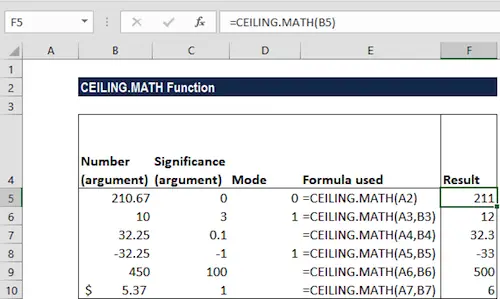# What Is The CEILING.MATH Function?

When you are looking to round numbers, one of the most common

functions that you need to use is the CEILING.MATH function.

Discover everything you need to know about rounding.

## What Is The CEILING.MATH Function?

Simply put, the CEILING.MATH Function is categorized under Excel Math and Trigonometry functions. It returns a number that is rounded up to the nearest integer or multiple of significance.

In the case of financial analysts, for example, the CEILING.MATH function can be used in setting the pricing after currency conversion, discounts, etc. When preparing financial models, it helps us round up the numbers as per the requirement.

## CEILING.MATH Function Formula

=CEILING.MATH(number, [significance], [mode])

The CEILING.MATH function uses the following arguments:

#1: Number (required argument) – This is the value that we wish to round off.

#2: Significance (optional argument) – This specifies the multiple of significance to round the supplied number to.

If you omit the argument, it takes the default value of 1. That is, it will round up to the nearest integer. Significance will ignore the arithmetic sign. Remember that by default, the significance argument is +1 for positive numbers and -1 for negative numbers.

#3: Mode (optional argument) – This will reverse the direction of rounding for negative numbers only.

• If the mode argument is equal to zero, negative numbers are rounded up towards zero.
• If the mode argument is equal to any other numeric value, negative numbers are rounded up away from zero.

Make sure to use our nearest hundred calculator.

## How To Use The CEILING.MATH Function In Excel?

To understand the uses of the CEILING.MATH function, let’s consider a few examples:

Example #1: Let’s see the results from the function when we provide the following data:

The formula used and results in MS Excel are shown in the screenshot below:

Example #2: Suppose we wish to know how many containers we will need to hold a given number of items. The data given to us is shown below:

The items per container indicate the number of items that can be held in a container.

The formula we will use is

=CEILING.MATH(A2,B2).

It rounds up A2 to the nearest multiple of B2 (that is items per container). The value derived will then be divided by the number of containers.

Learn how to use our round to the nearest hundredth calculator.

For example, in the second row:

=CEILING.MATH(385,24)/24, 385 will be rounded to a multiple of 24 and the result will be divided by 24.

We get the result below: